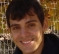## Fixed-point FFT scalingStarted by 6 years ago6 replieslatest reply 6 years ago517 views

Hi,

I have seen other discussions on this topic in this forum. But I am still not too clear on this.

Here is my understanding : Basically, the need for scaling FFT is because of the gain factor 'N'.

If we assume that my input is 16-bit, for a 256-point FFT implemented in radix-2 or radix-4, the gain factor is still N. Theoretically, if the internal datapath size was increased to 24-bits, there would be no need for any scaling. Right?

Now, increasing the datapath size to hold the final bit-width becomes too expensive as we increase the FFT size, hence we try to compensate for this gain and spread it across each of the stage/pass. For 256-point radix-2, which has 8 stages/passes, the gain should be scaled by 1/2 at each of them, right? Because gain of FFT is 256.

But I have seen it is mentioned 1/2 on every other pass in other forums. Why is this so?

FYI: Assume my input real and is 16-bits from sigma-delta+filter with single sinusoid tone. Which has a noise floor of about 70dB.

Thanks,

Krishna

[ - ]Although adding two fixed point numbers requires one more bit in the worst case, this does not usually happen at every stage. A worst case would be the DC signal of maximum amplitude.

However, if you are using a DSP processor with registers with a given number of bits (in contrast to building FFT hardware), then prescaling the input to avoid the worst case would in general destroy the SNR.

So, what is done is to use saturation arithmetic to avoid catastrophes, and try to keep the RMS signal amplitude constant from stage to stage. This would require scaling by the inverse square root of 2 on every pass, but you can cheat by scaling by half at every other stage.

Yes, such strategies have some risk of overflow, but you need to trade-off the risks against the loss of input dynamic range.

If you are rolling your own hardware, then by all means add an extra bit for each butterfly.

Y(J)S

[ - ]If we can assume that your real input tone frequency is never close to 0 Hz, we can say that you will not experience gains larger than N/2. The input power will be split in at last two bins. However, close to N/2 gain are likely to happen for low noise input real tone.

[ - ]Why is the gain dependent on my input tone?

[ - ]It depends on your input signal frequency because of the DFT scalloping loss. For rectangular time window, the scallop loss can be as high as 3.92 dB (or 0.6368 in amplitude gain). Check this paper for a detailed explanation (  https://www.utdallas.edu/~cpb021000/EE%204361/Grea...).

You can very scalloping loss in MatLab using

x = exp(1j*2*pi*5.5*(0:63)/64);

X=1/64*abs(fft(x));

plot(0:63, X)

Replace x generation for the below code, if you want to see the problem for a real signal.

x = sin(2*pi*5.5*(0:63)/64);

Replace x for exp(1j*2*pi*5*(0:63)/64) or sin(2*pi*5*(0:63)/64) to see the difference in the DFT result.

[ - ]